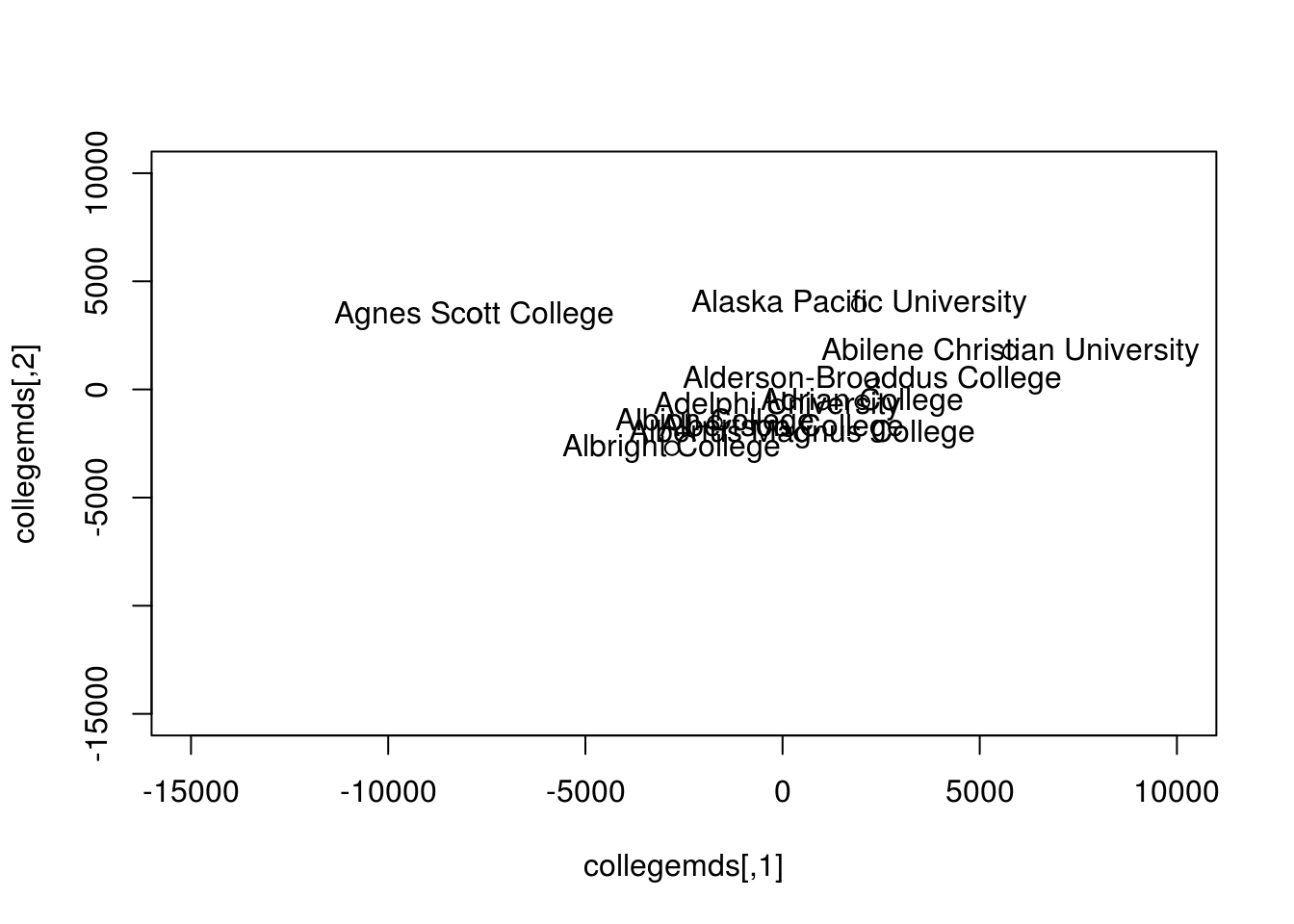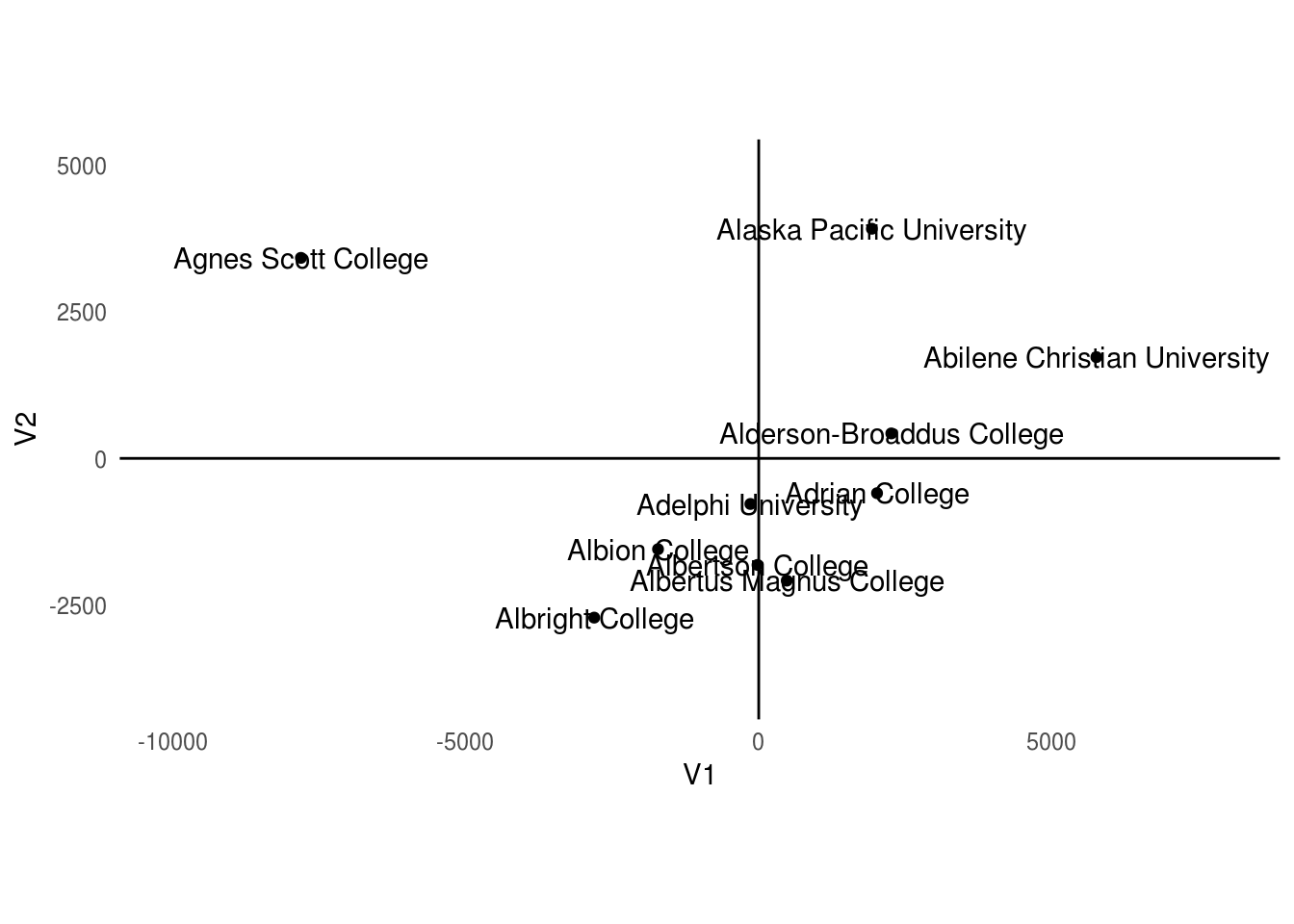# Multidimensional Scale in R

In this post, we will explore multidimensional scaling (MDS) in R. The main benefit of MDS is that it allows you to plot multivariate data into two dimensions. This allows you to create visuals of complex models. In addition, the plotting of MDS allows you to see relationships among examples in a dataset based on how far or close they are to each other.

We will use the “College” dataset from the “ISLR” package to create an MDS of the colleges that are in the data set. Below is some initial code.

``````library(ISLR);library(ggplot2)
data("College")
str(College)``````
``````## 'data.frame':    777 obs. of  18 variables:
##  \$ Private    : Factor w/ 2 levels "No","Yes": 2 2 2 2 2 2 2 2 2 2 ...
##  \$ Apps       : num  1660 2186 1428 417 193 ...
##  \$ Accept     : num  1232 1924 1097 349 146 ...
##  \$ Enroll     : num  721 512 336 137 55 158 103 489 227 172 ...
##  \$ Top10perc  : num  23 16 22 60 16 38 17 37 30 21 ...
##  \$ Top25perc  : num  52 29 50 89 44 62 45 68 63 44 ...
##  \$ F.Undergrad: num  2885 2683 1036 510 249 ...
##  \$ P.Undergrad: num  537 1227 99 63 869 ...
##  \$ Outstate   : num  7440 12280 11250 12960 7560 ...
##  \$ Room.Board : num  3300 6450 3750 5450 4120 ...
##  \$ Books      : num  450 750 400 450 800 500 500 450 300 660 ...
##  \$ Personal   : num  2200 1500 1165 875 1500 ...
##  \$ PhD        : num  70 29 53 92 76 67 90 89 79 40 ...
##  \$ Terminal   : num  78 30 66 97 72 73 93 100 84 41 ...
##  \$ S.F.Ratio  : num  18.1 12.2 12.9 7.7 11.9 9.4 11.5 13.7 11.3 11.5 ...
##  \$ perc.alumni: num  12 16 30 37 2 11 26 37 23 15 ...
##  \$ Expend     : num  7041 10527 8735 19016 10922 ...
##  \$ Grad.Rate  : num  60 56 54 59 15 55 63 73 80 52 ...``````

Data Preparation

After using the “str” function we know that we need to remove the variable “Private” because it is a factor and type of MDS we are doing can only accommodate numerical variables. After removing this variable we will then make a matrix using the “as.matrix” function. Once the matrix is ready we can use the “cmdscale” function to create the actual two-dimensional MDS. Another point to mention is that for the sake of simplicity, we are only going to use the first ten colleges in the dataset. The reason being that using all 722 will m ake it hard to understand the plots we will make. Below is the code.

``````collegedata<-as.matrix(College[,-1])
collegemds<-cmdscale(dist(collegedata[1:10,]))``````

Data Analysis

We can now make our initial plot. The xlim and ylim arguments had to be played with a little for the plot to display properly. In addition, the “text” function was used to provide additional information such as the names of the colleges.

``````plot(collegemds,xlim=c(-15000,10000),ylim=c(-15000,10000))
text(collegemds[,1],collegemds[,2],rownames(collegemds))``````From the plot, you can see that even with only ten names it is messy. The colleges are mostly clumped together which makes it difficult to interpret. We can plot this with a four quadrant graph using “ggplot2”. First, we need to convert the matrix that we create to a dataframe.

``collegemdsdf<-as.data.frame(collegemds)``

We are now ready to use “ggplot” to create the four quadrant plot.

``````p<-ggplot(collegemdsdf, aes(x=V1, y=V2)) +
geom_point() +
lims(x=c(-10000,8000),y=c(-4000,5000)) +
theme_minimal() +
coord_fixed() +
geom_vline(xintercept = 5) + geom_hline(yintercept = 5)+geom_text(aes(label=rownames(collegemdsdf)))
p+theme(panel.grid.major = element_blank(), panel.grid.minor = element_blank())``````We set the horizontal and vertical line at the x and y-intercept respectively. By doing this it is much easier to understand and interpret the graph. Agnes Scott College is way off to the left while Alaska Pacific University, Abilene Christian College, and even Alderson-Broaddus College are clump together. The rest of the colleges are straddling below the x-axis.

Conclusion

In this example, we took several variables and condense them to two dimensions. This is the primary benefit of MDS. It allows you to visualize was cannot be visualized normally. The visualizing allows you to see the structure of the data from which you can draw inferences.